﻿ Chalkboard With Mathematical Formula Stock Photo - Image of mathematics, blurred: 128447350

# Chalkboard with mathematical formula.

## Chalk board with mathematical formula - classroom

Photo Taken On: October 08th, 2018

More similar stock images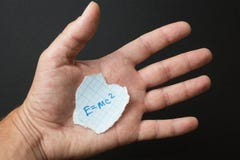The formula E = mc2 in the hand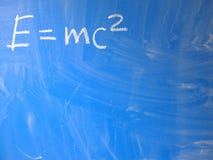Mathematical formula e=mc2 squared written on a blue, relatively dirty chalkboard by chalk. Located in the upper left corner of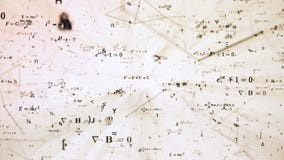Digital futuristic illustration with math, physics formulas in mesh network grid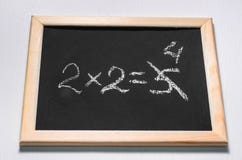A mathematical example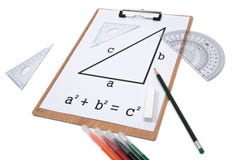Pythagorean Theorem.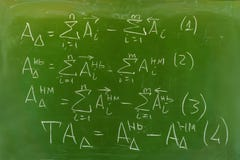Background - green chalkboard with hand-written formulas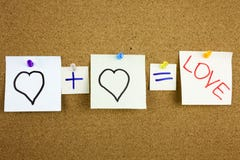A yellow sticky note post it writing, caption, inscription equation love or romantic relationship concept presented as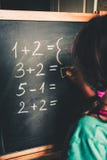Girl calculating by fingers to write the answer on chalkboard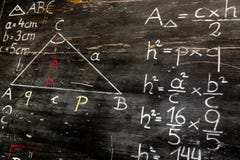Old blackboard with mathematical calculations and drawings
Related categories
Extended licenses